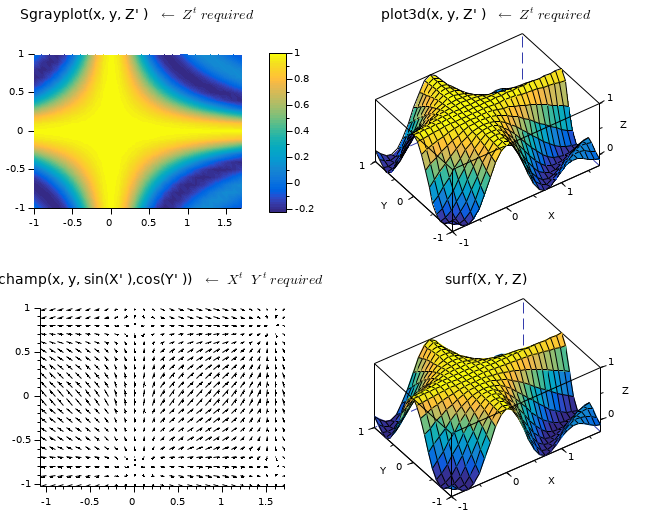Scilab Home page | Wiki | Bug tracker | Forge | Mailing list archives | ATOMS | File exchange
Change language to: English - Français - Português - Русский
Scilabヘルプ >> Elementary Functions > elementarymatrices > meshgrid

# meshgrid

Create matrices or 3-D arrays from vectors replicated in 2 or 3 dimensions

### Syntax

```U = meshgrid(u)
[U, V] = meshgrid(u)
[X, Y] = meshgrid(x, y)
[X, Y, Z] = meshgrid(x, y, z)```

### Arguments

u, x, y, z
Vectors of any types, of lengths nu, nx, ny, nz.

U, V
Square matrices of size nu x nu

X, Y, Z
Matrices or hypermatrices of the same size [ny, nx] or [ny, nx, nz].

### Description

meshgrid(..) builds matrices or 3-D hypermatrices by replicating input vectors along 2 or 3 dimensions.To create arrays of size [nx, ny] instead of [ny, nx], or with more than 3 dimensions, please use `ndgrid(x, y)` or `ndgrid(x, y, z,..)`.Most of Scilab 2D or 3D graphical functions like `champ()`, `grayplot()`, `Sgrayplot()`, `plot3d()`, `contour()`, etc.. work with grids generated with `ndgrid()`, not from `meshgrid()`. Data computed on grids coming from `meshgrid()` will require being transposed (please see the example thereafter).

### Examples

`[X, Y] = meshgrid(0:2)`
```--> [X, Y] = meshgrid(0:2)
X  =
0.   1.   2.
0.   1.   2.
0.   1.   2.

Y  =
0.   0.   0.
1.   1.   1.
2.   2.   2.
```

`[X, Y] = meshgrid(["a" "bc" "αβγδ"], [-3 5])`
```--> [X, Y] = meshgrid(["a" "bc" "αβγδ"], [-3 5])
X  =
!a  bc  αβγδ  !
!a  bc  αβγδ  !

Y  =
-3.  -3.  -3.
5.   5.   5.
```

Application to grids for graphics:

```// Generating data
x = -1:0.1:1.7;
y = -1:0.1:1;
[X, Y] = meshgrid(x, y);
Z = sinc(2*%pi * X.*Y);

// Setting the graphic figure
clf
drawlater
gcf().color_map = parulacolormap(64);
gcf().axes_size = [650 530];

// Plotting
subplot(2,2,1)
Sgrayplot(x, y, Z')
title(["Sgrayplot(x, y, Z'' ) " "\$←\;Z^t\; required\$"], "fontsize",3)
isoview, colorbar

subplot(2,2,2)
plot3d(x, y, Z', -125,50)
gce().color_flag = 1;
title(["plot3d(x, y, Z'' ) " "\$←\;Z^t\; required\$"], "fontsize",3)
isoview

subplot(2,2,3)
champ(x,y,sin(2*X'),cos(2*Y'))
title(["champ(x, y, sin(X'' ),cos(Y'' ))" "\$←\;X^t\;\;Y^t\; required\$"], "fontsize",3)
isoview

subplot(2,2,4)
surf(X, Y, Z)
title("surf(X, Y, Z)", "fontsize",3)
isoview

gcf().children.tight_limits = ["on" "on" "on"];
drawnow```• ndgrid — build matrices or N-D arrays by replicating some template vectors
• repmat — 配列を複製/タイル状に配置
• feval — 関数(または外部ルーチン)を評価する